CBSE Class 9 Triangles MCQ New

## CBSE Class 9 Triangles MCQ By Clarify Knowledge

CBSE Class 9 Triangles MCQ New Pattern 2022

## CBSE Class 9 Triangles MCQ Table

Question 1.
In given figure, the measure of ∠BAC is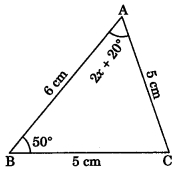(a) 60°
(b) 50°
(c) 70°
(d) 80°

Question 2.
In figure if AE || DC and AB = AC, find the value of ∠ABD.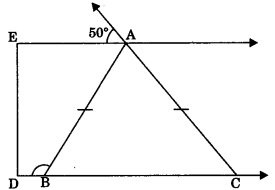(a) 130°
(b) 110°
(c) 120°

Question 3.
In figure X is a point in the interior of square ABCD, AXYZ is also a square. If DY = 3 cm and AZ = 2 cm, then find BY.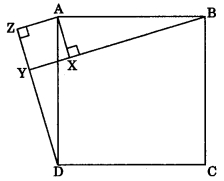(a) 5 cm
(b) 6 cm
(c) 7 cm

Question 4.
In figure AB ⊥ AE, BC ⊥ AB, CE = DE and ∠AED = 120°, then find ∠ECD.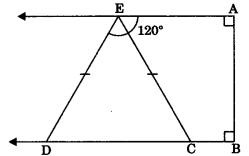(a) 80°
(b) 70°
(c) 85°

Question 5.
In ΔABC, ∠C = ∠A and BC = 4 cm and AC = 5 cm, then find length of AB.
(a) 5 cm
(b) 3 cm
(c) 4 cm

Question 6.
In ΔABC, AB = AC and ∠B = 50°, then find ∠C.
(a) 50°
(b) 40°
(c) 80°

Question 7.
In figure, D is the mid-point of side BC of a ΔABC and ∠ABD = 50°. If AD = BD = CD, then find the measure of ∠ACD.(a) 30°
(b) 70°
(c) 80°

Question 8.
In figure, ABC is an isosceles triangle whose side AC is produce to E and through C, CD is drawn parallel to BA. Find the value of x.(a) 52°
(b) 156°
(c) 76°

Question 9.
In figure AB ⊥ BE and EF ⊥ BE. If BC = DE and AB = EF, then ΔABD is congruent to(a) ΔEFC
(b) ΔECF
(c) ΔDEF

Question 10.
D is a point on the side BC of a ΔABC such that AD bisects ∠BAC. Then
(a) BD = CD
(b) BA > BD
(c) BD > BA

Question 11.
It is given that ΔABC = ΔFDE and AB = 5 cm, ∠B = 40° and ∠A = 80°. Then which of the following is true?
(а) DF = 5 cm, ∠F = 60°
(b) DF = 5 cm, ∠E = 60°
(c) DE = 5 cm, ∠E = 60°
(d) DE = 5 cm, ∠D = 40°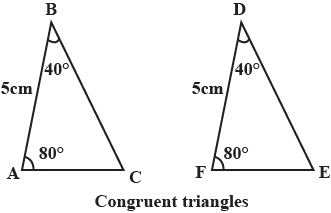Answer

Question 12.
Two sides of a triangle are of lengths 5 cm and 1.5 cm. The length of the third side of the triangle cannot be
(a) 3.6 cm
(b) 4.1 cm
(c) 3.8 cm

Question 13.
In ΔPQR, if ∠R > ∠Q, then
(a) QR > PR
(b) PQ > PR
(c) PQ < PR

Question 14.
In triangles ABC and PQR, AB = AC, ∠C = ∠P and ∠B = ∠Q. The two triangles are
(a) isosceles but not congruent
(b) isosceles and congruent
(c) congruent but not isosceles